## Saturday, March 12, 2016

### A common pitfall with correlations in timeseries

Correlations are often considered an important measure to understand the underlying (probably hidden) patterns in data sets. But as with other statistical measures, a complex situation (many variables, many rows of data) is reduced to a simple numeric value which might be problematic. For example, Ancombe’s quartet shows four totally different patterns of data with the same median, variance, correlation and linear regression.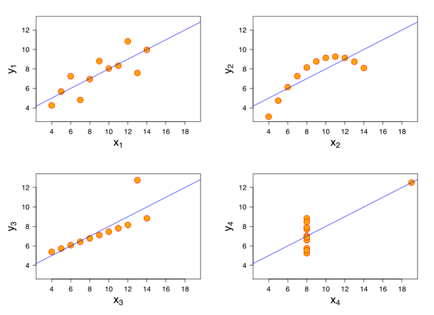Source: Wikipedia (https://en.wikipedia.org/wiki/Anscombe's_quartet)

Many articles about correlations also focus on the difference between correlations with and without causality. For example, there is a correlation between the sales of ice cream and the number of cases of sunburn. Having such a correlation allows to estimate one fact (e.g. cases of sunburn) with the observations of another fact (e.g. sales of ice cream). However, there is no direct causality between the two which means that you cannot control one fact with the other. For example, if you prohibit selling ice cream, you will not influence the cases of sunburn. The correlation however is still valid because the two fact share the same reason (hot temperature in summer because of high solar radiation). So, correlations can be divided in at least three classes:

• causality (A => B)
• correlation with a common reason (there exists a C with C=>A and C=>B and as a result A correlates with B)
• correlation without a common reason / accidental correlations (there doesn’t exist any C with C=>A and C=>B and the correlation between A and B is purely random and not reliable)

While the first two are a reliable source for detecting patterns in data sets, the accidental correlations might lead to wrong results. You can find a lot of strange and even funny correlations, for example on this website (divorce rate in Main perfectly correlates with the consumption of margarine). Another very prominent example is the correlation between media mentions of Jennifer Lowrence and the Dow Jones, which was explained by Tom Fawcett in his article “Avoiding common mistakes with time series”: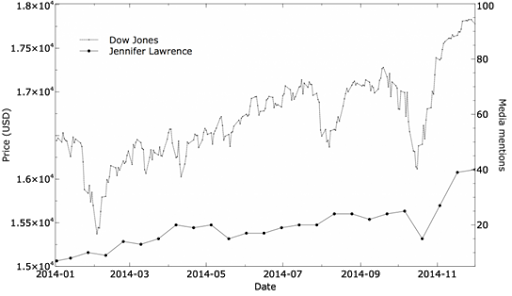As a consequence, we need to avoid these false correlations. But do these correlation appear likely or are they a very rare observation? Well, the sheer amount of internet sources showing funny examples as the one above could indicate that false correlations are not unlikely to happen.

In order to investigate this, I used generated random walk processes based on normally distributed random values. Random walk processes are processes were the random variable is the delta of two points (not the absolute value of the point). Many real life processes can be described as random walk processes, for example temperature (for machine sensors or for weather data), birth rates, even sales (at least to some extend) etc., so these processes are likely to be seen when analyzing time series data.

The setup for the experiment was to generate 10 variables with 100 observations each. Here is an example: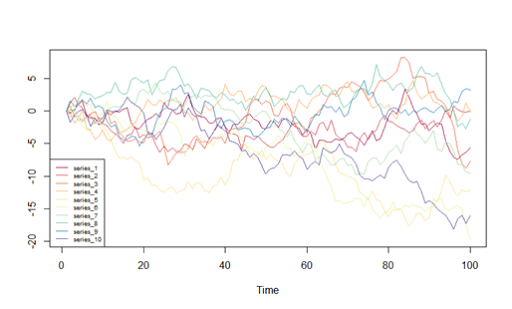Next, I calculated the correlation matrix for the 10 variables to find strong positive/negative correlation: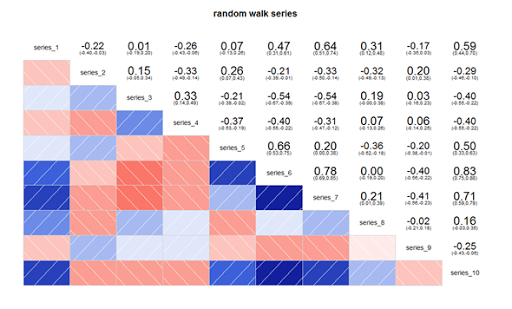As you can see we have some series with a strong correlation, especially with a strong positive correlation (deep blue = series 6 and series 10 with a correlation of .83) in this example. Plotting the series with the strong correlation looks like this: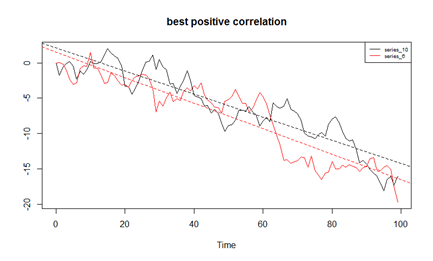Of course, not every combination correlates well, for example series 6 and 8 have a correlation near zero. But in this example with just 10 variables we found 2 with a good correlation although the series were created randomly. Since this was just a single random experiment with no statistical relevance, I repeated the experiment 100,000 times (Monte Carlo). The random number generator was Mersenne Twister, normally distributed random variables have been computed using Box-Muller. Here is the distribution of the best absolute correlation between two variables in each experiment: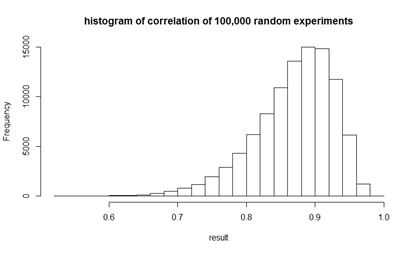It may be surprising that such a majority of our random experiments ended with at least two series with a good correlation. The result shows that in 99% of the experiments the best correlation was at least .7, in 88% it was at least .8 and in 34% at was at least .9.

This means that with a confidence of almost 90% we can expect a correlation of .8 for this random experiment or in other words, it is very likely to find a correlation of 10 random walk processes (with 100 observations each). This also means, that when analyzing data you should not blindly celebrate any correlation being found but in the opposite be very skeptical about correlations in your data. You might argue that this effect is a result of our relative short time series with only 100 values (“rows”) for 10 given variables. However, repeating the test for 10,000 values per variable gives exactly the same result. The most important influence is the number of variables. While the correlation is rather poor with 2 or 3 variables, you can be almost absolutely sure to find a correlation of above .8 with 25 variables as shown in the table and chart below (black line: Correlation of .8 and above, the shaded area shows the range from correlation .7 to .9).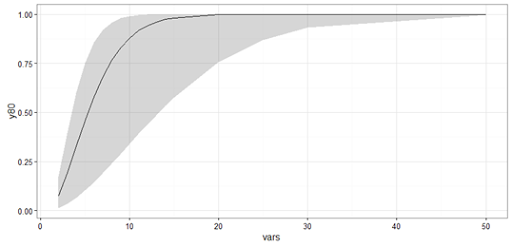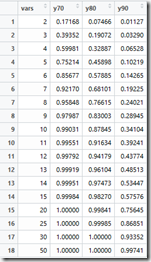Conclusion

Correlations of variables in data sets are often put on the same level with meaning. One may think to have found a hidden pattern when a correlation is detected. However, this post shows that correlations are not rare, but are very likely to be discovered even in randomized data sets with only 10 variables. As a result, we need to carefully examine each correlation we find in a data set. A first step can be to use a holdout and to test the discovered correlations on the holdout data set (much like we use training and testing data sets in data mining).